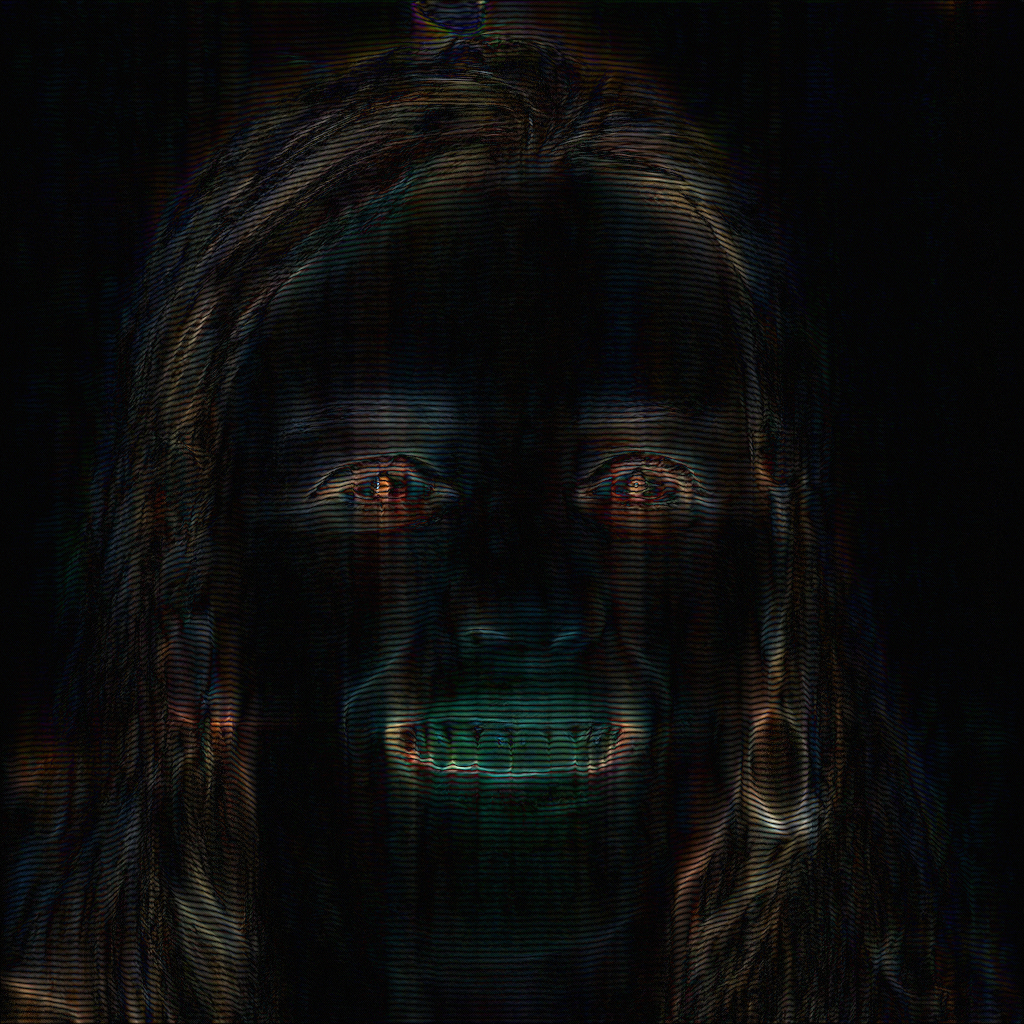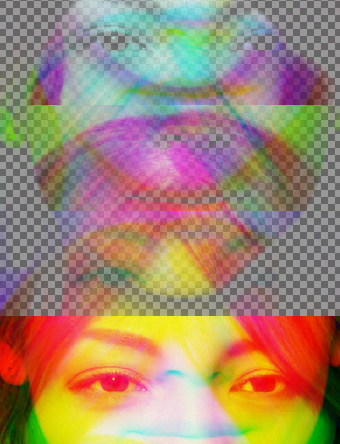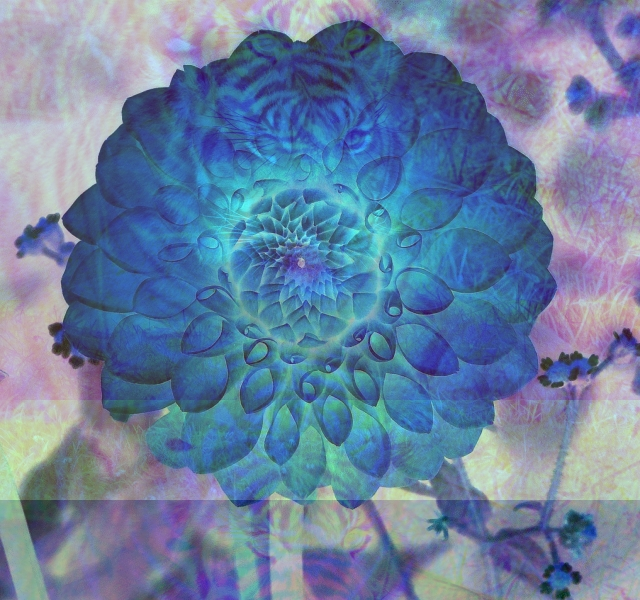# Glitch Art Filters?

Two new scripts, both based on DCT operations. The first one messes with the DCT transforms of entire images, while the second is another take on that JFIF one I was previously focussing on with a few new features.(Edited) Updated the new JFIF script again, along with subsampling it now handles quality slightly differently. It also has the glitch punch card thing implemented but I’m not sure how to get it to work in the way that it should where the locations of broken blocks coincide for each channel. I was able to do this with the older script but I don’t yet know how to do it for this one.

https://pastebin.com/K53DFtaQ

@Joan_Rake1 You wouldn’t happen to know how to index square areas of image to #colors, would you? I’m gonna need it to update my transfer color for better stimulation of older systems. There’s also arbitrary stimulations I found in an android app named 8-bit photo lab.

There’s already the quadtree variations script and the posterise one too. Try modifying those.

@Joan_Rake1 Finally got around to testing the filters. Let’s see…

JFIF Effect 2 : With 32 GB of RAM - It takes me over a minute to finish. I prefer your original filter, much faster, and it works. I think I"ll past on this one.

DCT FSU on the other hand. I find it useful though hard to get a nice image out of it, but the lines may have some applications though I just can’t figure out its application.

EDIT: Figured out the DCT FSU, I really like how the patterns looks like. It could work well with blending modes on the final version.

Here’s a test image of DCT FSU using a non-existent person -

By the way, I am attempting to make a cli command where you can apply commands per square of specified size. That would be good for glitch art, and it would be good for my pixel art command as many hardware have color restriction based on size.

And I just figured it out though it requires one to resize canvas, create a loop to keep certain areas, rotate all of those looped generated image, and apply the keep effect loop for those image. Then rotate them all again, and then assign your desired filter, and then append them altogether. That doesn’t sound so enjoyable to do. And I’m failing to resize by canvas rather than the image though I have a workaround using f"" to replicate the original image, and using w,h,depth,channel.

Also, I can’t seem to figure out how to keep a variable constant. Like iw = w, I want it to remain based on the original image.

Remarks

1. If there is repeated rotation, you might not need to rotate so many times; only decide what the final position is, then rotate once.
2. If you need some sort of tiling, you have `apply_tiles`.
3. There is something called global variables. There is a large section on variables in the reference documentation.

Apply_tiles actually worked for me. Thanks. If I didn’t knew of apply tiles, then I would have a headache making the cli and going all the way to doing unnecessary work. Well, time for me to add stimulate hardware restriction i.e few colors per tiles on reduced colors transfer. I’ll keep in mind about global variables using the reference documentation when I’m ready to use them.

@Joan_Rake1 - Any updates on those filters?

I just found this. I knew that fill can break something in a interesting manner. This “glitch” might be explored.

The command is very simple

autocrop f. .Result via preview window. Now, that is interesting.

Could literally just have a random layer, and then keep the filtered layer only. That’s all you need here. Previews isn’t to be trusted though.

Could you upload your input image? Nothing happens when I do it to `sample tiger` for instance…

I forgot to mention that there needs to be a dot next to first . Also, second layer needs to have something.

I don’t know what to do, so I did something different.

``````gmic sample tiger,flower autocrop_seq auto +local autocrop fill. . endlocal -
``````PS With `sample colorful,tulips`

The result would look way better with transparent image on layer 2.

Here’s something of interest, what is this image for and for what PDN glitch does it relate to?

Hint - What it’s in the left? The right is a search pattern, and the result is in the left.

Made this as a reference for coding that glitch. Not really helping so much, but better G’MIC coders can figure this out quicker.

I knew that fill can break something in a interesting manner.

That’s something to do with the dimensions of the image changing while the number of pixels being used doesn’t. The fill command seems to prefer pixel indices rather than actual locations within the image. Here’s one way to replicate the effect:

``````ww={w}
hh={h}
dimx={int(100)}
dimy={int(\$ww)}
s c s z
unroll x resize \$dimx,\$dimy,100%,100%,-1
a c a z
``````

Using this method it’s possible to change the order of the pixels to get different patterns as well as through changing the final dimensions.

1 Like

I’m thinking that I should add peak filters and resonance to my Butterworth Bandpass filter so that I can turn it into a full equaliser of sorts. However, resonance doesn’t seem so easy to add since I’m using filters which have real-valued orders instead of integer-valued orders and I don’t want to sacrifice that feature. The only explanation that I’ve found on how to create such monsters is on some StackExchange site.

I’m interested in the gain and not so much the phase, though I could add that later. I don’t see a gain function there, and the only function that I do see looks like it’s second-order. I’m no maths whizz so I’ll need some pointers on what to do, but I can try to summarise what I want: given the relative gain function, where should the Q term go?

Edit: I’ve not only figured that out but I’ve built a 5-band-shelf EQ with the LP and HP from before. It’s very rough though and it could be made a bit faster. I have to sort out how the frequencies scale with the image size but I’m a bit stuck on how to do it. https://pastebin.com/WkXivbuc

Suggestion on scaling the frequency to image size. Maybe have a “surface” function where x=0 is treated as 0 and width is treated as 1. If the dimensions are different, then that should be taken care of by multiplying by min(w,h)/max(w,h). But before you do all of that, there should be a condition to test which is bigger, so that one of them is 0,1 range. That way, the frequency will scale within the image dimensions.

f “s/d=min(w,h)/max(w,h);
norm_w=w>h?x/w-.5:(x/w-.5)*sd;
norm_h=w>h?(y/h-.5)*sd:y/h-.5;”

Note: Quick write-up, not sure if it’ll work.

Also, I think I have shown you distortion cli command I was working on. They scale just fine. The reason being is that the base dimension to be used is always 1024 unless specified otherwise.

``````#@cli rep_logpindis: (eq. to rep_logarithmic_pinching_distortion)
rep_logpindis: rep_logarithmic_pinching_distortion \$*
#@cli rep_logarithmic_pinching_distortion: _distortion_main>=0,-1<=_preshift_x<=1,-1<=_preshift_y<=1,-1<=_effposition_x<=1,-1<=_effposition_y<=1,_prescale_x,_prescale_y,_afterscale_x,_afterscale_y,0<=_angle<=360,_mirror_placement= { 0=outside-in | 1=inside-out },_lx_axis= { 0=different axis | 1=same axis},_ly_axis= { 0=different axis | 1=same axis},ix_dir={ 0=negative | 1=positive },iy_dir={ 0=negative | 1=positive },_boundary= { 0=Periodic | 1=Mirror },1<=_interpolation<=5,_dimension_ref>0
rep_logarithmic_pinching_distortion:
skip \${2=0},\${3=0},\${4=0},\${5=0},\${6=1},\${7=1},\${8=1},\${9=1},\${10=0},\${11=0},\${12=1},\${13=0},\${14=1},\${15=0},\${16=0},\${17=5},\${18=1024}
if \$6==0||\$7==0||\$8==0||\$9==0 v + error "Scale cannot be 0!" v - fi
if \$17<1 v + error "interpolation cannot be less than 1" v - fi

shift {50*\$2}%,{50*\$3}%,0,0,3,1
f "
sd=w/h;
ang=pi*(\$10/180);
ox=\$4*-1;
oy=\$5;
ox/=h>w?1/sd:1;
oy/=w>h?sd:1;
OX=ox*cos(ang)-oy*sin(ang);
OY=ox*sin(ang)+oy*cos(ang);
ox=OX;
oy=OY;
ix=((x/w)-.5)/(h>w?1/sd:1);
iy=((y/h)-.5)/(w>h?sd:1);
IX=ix*cos(ang)-iy*sin(ang);
IY=ix*sin(ang)+iy*cos(ang);
ix=IX;
iy=IY;
ix=abs(ix+ox/2)*\$6;
iy=abs(iy+oy/2)*\$7;
ed=int(abs(\$18));
ed/=2;
ix*=ed;
iy*=ed;
lx=ix==0||iy==0?log(10^-8)*\$1:log(\$12?iy^2:ix*iy)*\$1;
ly=iy==0||iy==0?log(10^-8)*\$1:log(\$13?ix^2:ix*iy)*\$1;
dx=(\$14?1:-1);
dy=(\$15?1:-1);
ix-=lx*dx;
iy-=ly*dy;
ix*=w/ed;
iy*=h/ed;
ix*=\$8;
iy*=\$9;
i(ix,iy,z,c,\$17,(\$16?3:2));
"
if \$11 shift 50%,50%,0,0,3,1 fi
``````

I’m unclear on how that specific script would work in the context of an EQ like this. What I’m trying to do is express the frequencies of the FFT being used for the EQ in a logarithmic format. The parameters for the frequency are between 0 and 16, but this must be scaled to match the whole of the image so that one can work with the highest frequencies and I’m struggling to see how it would be done.

On another note, here’s two attempts at rebuilding a JFIF glitch script. They’re very slow despite the DCT tiles being 40x40. I know that David’s JPEG artefacts script uses a preloaded image for the tiles which correspond to different frequencies, which is much quicker. The issue with this is that it only works for preset tile sizes. Should I generate the tiles first and then draw them on in the way that David’s script does, and if so, how can I generate them?

``````to_rgb
csswap 0,14
w,h={[w,h]} r {w+(-w%40)},{h+(-h%40)},1,100%,0,3
ww={w}
hh={h}
minl=0.05*wh
maxl=0.1*wh
split_tiles -40,-40
dct f "I*cut(10-(x+y)/2,0,1)"
append_tiles {\$ww/40}
f ">begin(const minl="\$minl";const maxl="\$maxl";cd=round(u(minl,maxl)));
cd-=1;
(cd<=0)?(cd=round(u(minl,maxl));J=[u*50000,u*50000,u*50000]):(J)"
split_tiles -40,-40
idct
append_tiles {\$ww/40}
csswap 14,0

##############################

to_rgb
csswap 0,14
w,h={[w,h]} r {w+(-w%40)},{h+(-h%40)},1,100%,0,3
minl=0.05*wh
maxl=0.1*wh
at "dct f ""I*cut(10-(x+y)/2,0,1)""",40,40,100%,0,0,0,3
f ">begin(const minl="\$minl";const maxl="\$maxl";cd=round(u(minl,maxl)));
cd-=1;
(cd<=0)?(cd=round(u(minl,maxl));J=[u*50000,u*50000,u*50000]):(J)"
at "idct",40,40,100%,0,0,0,3
csswap 14,0
``````

On a tangential note, I’ve updated my Satellite command and I’m gonna put my take on some of the IR window colour schemes here. irwindow.txt (1.7 KB)